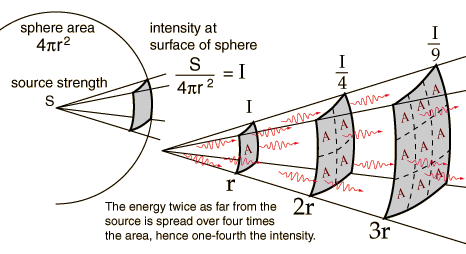# X is for x ray: Inverse square law and PID/cone length

This is the last week of post topics from the review for the hygiene written boards.  Today is the inverse square law and how this applies to changing the PID/cone length and exposure settings for a comparable image density.

### Inverse square law

The inverse square law applies to any source where the x (material, energy, etc.) being emitted goes in all directions.  This applies to x rays as they are emitted in all directions when they are produced.  It states that “The intensity of the influence at any given radius r is the source strength divided by the area of the sphere” (Georgia State University).  See diagram below.What this means is that if you are at a distance (r) from the source when that distance is doubled (2r) there is 1/4 the amount of radiation at this distance.  And when that distance is tripled (3r), there is 1/9 the amount of radiation at this distance.

### Inverse square law and PID/cone length

How the inverse square law comes into play is changing the PID/cone length of an x ray unit.  For example, changing the PID/cone length from 8 inches to 16 inches doubles the initial distance (2r).  This means that there is 1/4 the radiation exiting from the 16 inch PID/cone versus the 8 inch PID/cone.  To ensure your image density stays the same with this longer PID/cone, you would need to increase the exposure time 4 times.  If the PID was increased by 3x, such as a 24 inch PID/cone (I’m not aware that these exist but for learning purposes I’m using it 😀 ) this would be 1/9 the amount of radiation exiting the 24 inch PID/cone.  For comparable image density the time would need to be increased 9 times.

### Take home points

Double (2r) PID/cone length = Increase exposure time 4 times

Triple (3r) PID/cone length = Increase exposure time 9 times

Should you have any questions or thoughts on this topic (I realize its a little more complex than some of the last few), please let me know.

Thanks and enjoy!